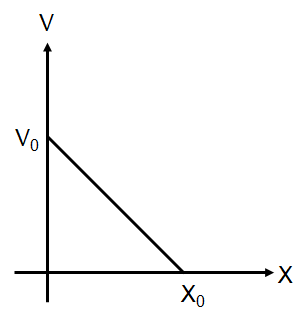Significant figures
Question

# The given graph shows the variation of velocity with displacement. Which one of the graph given below correctly represents the variation of acceleration with displacementModerate
Solution

## $\mathrm{From} \mathrm{graph} \mathrm{V}=-\frac{{\mathrm{V}}_{0}}{{\mathrm{x}}_{0}}\mathrm{x}+{\mathrm{V}}_{0}$$\mathrm{a}=\frac{\mathrm{dV}}{\mathrm{dt}}=-\frac{{\mathrm{V}}_{0}}{{\mathrm{x}}_{0}}\left(\frac{\mathrm{dx}}{\mathrm{dt}}\right)=-\frac{{\mathrm{V}}_{0}}{{\mathrm{x}}_{0}}\left(-\frac{{\mathrm{V}}_{0}}{{\mathrm{x}}_{0}}\mathrm{x}+{\mathrm{V}}_{0}\right)=\frac{{\mathrm{V}}_{0}^{2}}{{\mathrm{x}}_{0}^{2}}\mathrm{x}-\frac{{\mathrm{V}}_{0}^{2}}{{\mathrm{x}}_{0}}$

Get Instant Solutions